Statistical Mechanics homework Help at TutorEye

# Best Homework Help For Statistical Mechanics

## Statistical Mechanics:

Statistical Mechanics deals with the microscopic entities, where statistical methods and probability theories are applied to study the behaviour of atoms and molecules. It encompasses the laws of classical and quantum mechanics in order to measure the microscopic constituents of the particle. It is implied and drawn majorly with thermal energy of the system in disordered states and derived on the laws of probability and average behaviour of a large number of particles of the same kind.

## Statistical Mechanics Sample Questions:

Question 1: Maxwell-Boltzmann statistics can be applied applied to ________

a) atoms                            b) molecules
c) lattice                            d) all of the above

Answer: d) all of the above

Explanation: Maxwell-Boltzmann can be applied to only distinguishable particles. Atoms, molecules and lattices are distinguishable particles.

Question 2: At most probable speed, the density of molecules is __________.

a) minimum                           b) maximum
c) zero                                   d) infinity

Explanation: Most of the particles move with the most probable speed. As the velocity further increases, the density of molecules moving with that particular speed decreases.

Question 3: What is the average kinetic energy associated with each degree of freedom?

a) kT                              b) 2kT
c) kT/2                           d) kT/4

Explanation: According to the law of equipartition of energy, the average kinetic energy associated with each degree of freedom is kT/2.

Question 4: The ratio of the rms velocity to most probable velocity is ______.

a)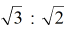b)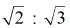c)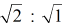d)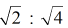Answer: a)Explanation: The equation of rms and most probable velocity is derived from Maxwell's speed distribution law.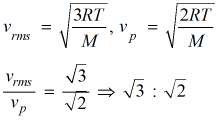Question 5: What would be the most probable velocity for one Oxygen molecule at 300 K?

a) 512 m/s                   b) 714 m/s
c) 812 m/s                   d) 981 m/s

Explanation: Mass of one oxygen molecule = 32g/mol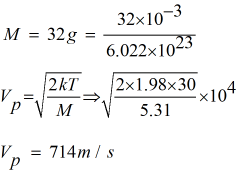Question 6: What is the Kinetic energy of 2g of oxygen at -23°C ?

a) 204.56 J                b) 256.87 J
c) 194.86 J                d) 176.89 J

Explanation: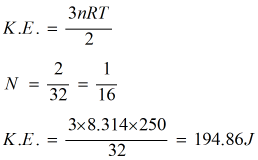Question 7: Kinetic energy of particles is dependent on ___________ only.

a) Pressure                     b) Velocity
c) Volume                       d) Temperature

Explanation: The kinetic energy of particle 3RT/2 is dependent only on the temperature. The increase in pressure is compensated by the corresponding decrease in the volume.

Question 8: What is the effect on the molecules moving with lower speed with increase in the temperature?

a) Decreases                     b) Increases
c) Same                             d) None of these

Explanation: As the temperature is increased, the number of molecules traveling with lower speed decreases whereas those traveling with higher speed increases.

Question 9: Phase space is a __________ dimensional space.

a) three                          b) four
c) six                              d) eight

Explanation: Phase space is a 6 dimensional space, consisting of the position x, y and z directions and its momentum in these directions.

Question 10: The speed at which most of the particles are moving is represented by ___________.

a) most probable speed                    b) rms speed
c) mean velocity                                d) none of these

Explanation: Most probable speed is the speed at which most particles are moving. It amounts to the speed at which highest fraction of molecules are moving.